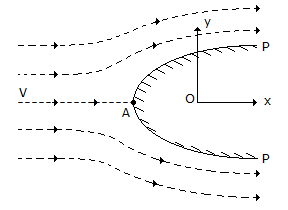# Civil Engineering - UPSC Civil Service Exam Questions

31.

The Chezy's coefficient C is related to Darcy-Weisbach friction factor f as

 A. C = (g/8f) B. C = (6g/f) C. C = (8g/f) D. C = (f/8g)

Explanation:

No answer description available for this question. Let us discuss.

32.

What is the maximum permissible longitudinal pitch in staggered riveted compression joints ?

 A. 500 mm B. 400 mm C. 300 mm D. 100 mm

Explanation:

No answer description available for this question. Let us discuss.

33.

For determining the forces in the members of a truss by graphic statics, the method of substitution will be required in the case of

 A. Fink truss B. Howe truss C. King post truss D. Warren truss

Explanation:

No answer description available for this question. Let us discuss.

34.

A Rankine (oval) half-body PP is subjected to a two-dimen-sional flow (x, y coordinate directions from origin O) with uniform velocity V, resulting in typical streamlines as shown in the figure by dotted lines. The point A on the body surface isA. separation point B. stall point C. stagnation point D. point of maximum velocity

Explanation:

No answer description available for this question. Let us discuss.

35.

A projectile is fired horizontally with a velocity of 6 m/s from a point of height h m above and 12 m away from an object. What is the value of h required so that projectile hits the object ?

 A. 4.9 m B. 9.8 m C. 6 m D. 19.6 m